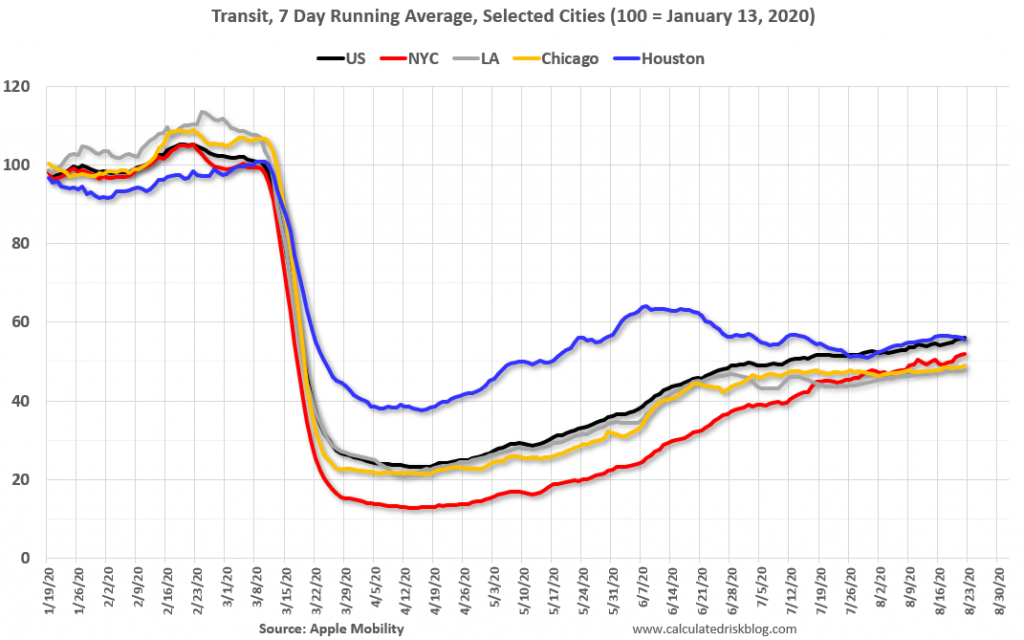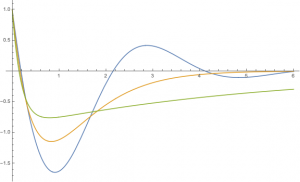# damping covidThis plot, from the Apple mobility site, reminded me of the standard paradigm of linear controlled systems: playing with your gains in linear control systems can affect damping behavior of your system.

It is intuitively clear from the picture, that something separates the NYC, Chicago, LA from Houston: one sees oscillations in Houston, not in the other three metropolises, – so reminiscent of the transition from overdamped to underdamped systems.

What is it? Here’s a toy model which accounts for this phenomenon. I will deal in hugely exaggerated generalities, interpreting the mobility data as a proxy for the state of the economic activity, impacted by the prevalence of the infection in the society. Correspondingly, I consider a coupled system consisting of Economy and Virus.

We assume that the virus growth is facilitated by the economy: the larger the economy, the more interactions between people, the faster the growth.

Economy, on the other hand, is stationary (one could insert a growth term, but it is far slower than that of virus, so we safely drop it), but is slowed down by the virus. (It should be noted, that our coordinate chart is centered around a presumed equilibrium, – so the negative “Economy” just means  below that equilibrium point etc.)

This slowdown by the virus is describing the natural, intrinsic reaction of the economy to the pandemic, such as the resources reallocated to the hospitals, workers skipping work because of sickness or need to help someone sick, supply chain disruption etc.

Independently pf that, there might be some control measures that the government or the population could undertake, – represents by the $$u$$ term.

As I am teaching a basic linear control course, all interactions here are assumed linear:

$\begin{array}{rcl}\dot{E}&=&-bV+u\\ \dot{V}&=&cE+dV.\\ \end{array}$

OK, what do we want? Obviously, to stabilize the system, keeping $$V$$ bounded. And $$E$$, too, – if the epidemics expands, the economy drops exponentially.

What our feedback control should be?

First instinct is, of course, to base the feedback entirely on the virus, $$u=-lV$$. However, this does not affect the trace of the resulting linear system (leaving it at $$d$$), and therefore is not enough for the stabilization.

One is forced to have a negative feedback based on $$E$$: shutdowns are necessary for the stabilization!

So, accepting that, take $$u=-kE-lV$$, resulting in

$\begin{array}{rcl}\dot{E}&=&-kE-(l+b)V\\ \dot{V}&=&cE+dV.\\ \end{array}.$

To simplify the analysis, note that by rescaling $$E,V$$ (who cares how we count the critters, – by trillions or by micrograms?) we can make $$b$$ and $$c$$ equal, and by changing the time units  we can make them both equal to $$1$$.  So, the system reduces to

$\begin{array}{rcl}\dot{E}&=&-kE-(l+1)V\\ \dot{V}&=&E+dV.\\ \end{array}.$

When it is stable? Well, when the trace and the determinant of the RHS matrix are negative and positive, respectively, i.e. when

$k>d, \mbox{ and } l+1>kd.$

Those are the conditions of stability!

Ramping up the unseriousness of this model, we assume now that the gain $$l$$ is not really in government hands, – it represents the reaction to virus spread that the governments (at least in this polity) are too inept to control. In our model, $$l$$ the public’s reactions to what it observes about the virus spread. So we will leave it fixed, and will have a look at what the government can tune up, –  the gain $$k$$. The larger $$k$$, the more severe the government lockup are.

What gain $$k$$ yields the stable system? well, it should be found in the interval
$d\leq k\leq \frac{l+1}{d}.$
What is the best value there? One natural marker is the value where the eigenvalues of the RHS operator (“poles” in the control theory parlance) merge, which happens at
$k_*=2\sqrt{l+1}-d\leq \frac{l+1}{d}.$Below this level, oscillations start, above, the system is overdamped (good), but the decay rate can be improved.

Here are the plots of a family of the solutions with $$d=1/2, l=3$$ and $$k=1.6, 3.5, 6$$… Orange is at the critical level $$k=3.5$$; green well above, blue, well below.

One can see clear resemblance with the plots above…

This model is just a toy, created to illustrate implicit feedback structures built into our societal decisions, not to produce some specific recommendations (Houston, your $$k$$ is too low!)… And yet. We rarely think about governance in control-theoretic terms, but we should. In particular, control theory should govern our anticyclical macroeconomic responses, such as the levels of unemployment benefits.

Control theory is old, but its usefulness is still underestimated.